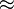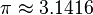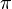# Approximation facts for kids

Kids Encyclopedia Facts

An approximation is a version of a piece of information that does not describe it exactly, but is close enough to be used. An approximation may be used either when the exact piece of information is not known, or when it's too long or complicated and people need something simpler.

For example, if you wanted to add 2.0002 and 2.0003, you can add 2 and 2, and it would be an approximation. If you are left with a balance of Dollar 78.23 you might want to say you are left with 80 dollars. In mathematics, this is written using the$\approx$ symbol (e.g.,$\pi \approx 3.1416$).

Archimedes used polygons to approximate a circle, and to calculate an approximation for$\pi$ as shown below:

## Related pagesApproximation Facts for Kids. Kiddle Encyclopedia.